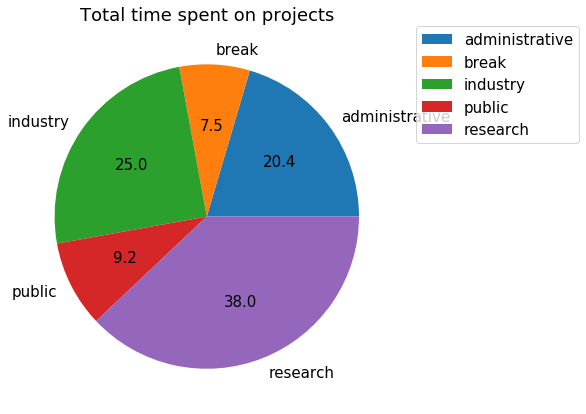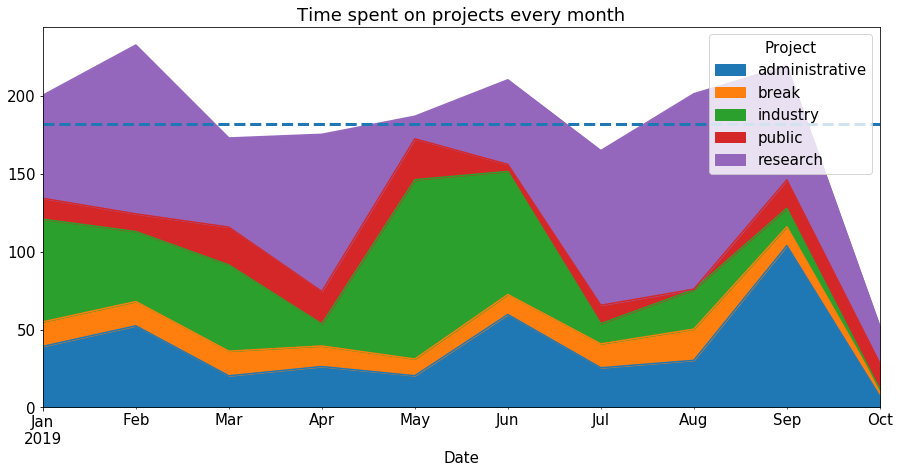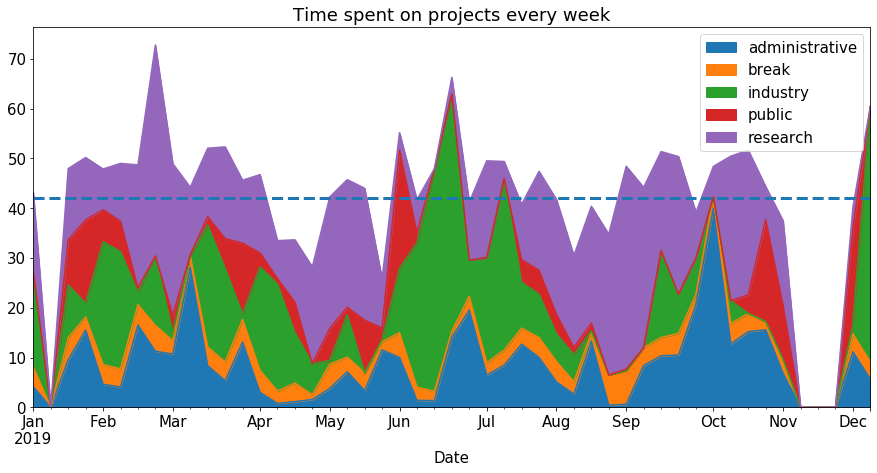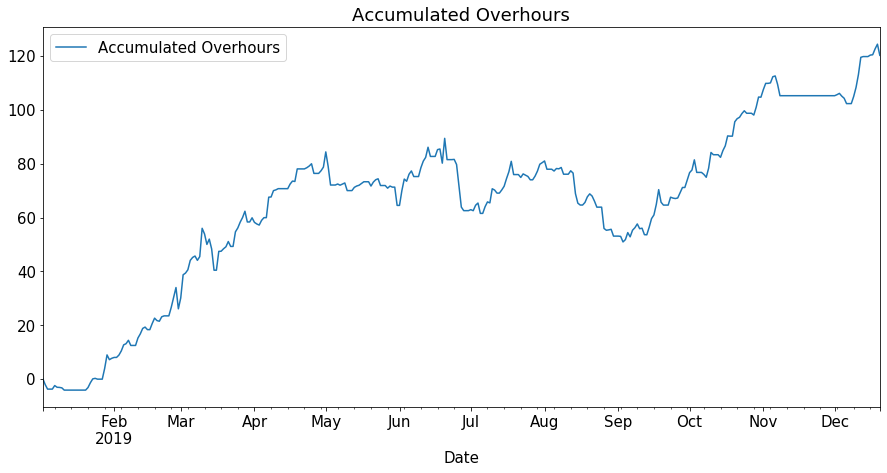# Gleeo time plotter

Published:

I have been using the Gleeo time tracker app on my phone to track my time at work for multiple years. To make sense of the data, I implemented a jupyter notebook. The notebook reports:

• Total time spent per project
• Time spent per project [per unit of time]
• Over(under)hours aggregation, accounting for holidays

You can fork the jupyter notebook and try it with your own data.

# The notebook

This is what the notebook outputs with some scrambled time tracking data for 2019. We first read the Time Tracker data export.csv file with pandas, which you can export from the Gleeo app:

import pandas as pd
import numpy as np
time_entries = pd.read_csv("Time Tracker data export.csv")
time_entries['Start'] = pd.to_datetime(time_entries['Start'])
time_entries['End'] = pd.to_datetime(time_entries['End'])
projects = time_entries['Project'].unique()
time_entries

1workresearchwritingNaN2019-01-02 09:38:00...2.766667NaNNaNNaNNaN
2otherbreaklunchNaN2019-01-02 12:24:00...0.683333NaNNaNNaNNaN
3workresearchwritingNaN2019-01-02 13:05:00...5.033333NaNNaNNaNNaN
....................................
1506workresearchhardwareNaN2019-12-20 09:14:00...0.266667NaNNaNNaNNaN
1508workresearchhardwareNaN2019-12-20 10:35:00...1.666667NaNNaNNaNNaN
1509otherbreaklunchNaN2019-12-20 12:15:00...1.083333NaNNaNNaNNaN
1510workresearchhardwareNaN2019-12-20 13:20:00...0.733333NaNNaNNaNNaN

1511 rows × 13 columns

## Total time spent on projects

Let’s print the total time spent on projects:

project_total = time_entries.groupby('Project').sum()
project_total["Decimal Duration"]


All times are displayed in hours:

Project
break             158.566667
industry          530.433333
public            195.533333
research          806.933333
Name: Decimal Duration, dtype: float64


Proportion of time spent on projects:

ax = project_total.plot.pie(y='Decimal Duration', autopct='%.1f')
ax.set_title('Total time spent on projects')
ax.set_ylabel('')## Time spent on projects per unit of time

Let’s plot the time spent on project over time of the year. We define a function that reorganizes the tracked time in regular time interval:

start = time_entries.iloc['Start'].round('D')
end = time_entries.iloc[-1]['End'].round('D')
years = range(start.year, end.year+1)

def get_time_spent_per(time_unit):
plt_time_index = pd.date_range(start, end, freq = time_unit)
range_df = pd.DataFrame(plt_time_index, index=plt_time_index, columns=['Date'])
range_df['End'] = range_df['Date'].shift(-1)
range_df = range_df.iloc[:-1] # drop last range

series = [ time_entries.loc[(time_entries['Start'] > row['Date']) & (time_entries['End'] < row['End'])]\
.groupby('Project')['Decimal Duration'].sum()
for _, row in range_df.iterrows() ]
organized_durations = pd.DataFrame(series, index=range_df['Date']).fillna(0)
return organized_durations


We can use this function to plot the time spent on project per month:

df_month = get_time_spent_per("BM")
ax = df_month.plot.area(title="Time spent on projects every month")
average_hours_per_month = df_month.mean().sum()
print("Average hours/month: {}".format(average_hours_per_month))
ax.axhline(average_hours_per_month, linestyle="--", linewidth=3)


The average hours/months for all projects (including the break project):

Average hours/month: 181.71833333333333Or per week:

df_week = get_time_spent_per("W")
ax = df_week.plot.area(title="Time spent on projects every week")
average_hours_per_week = df_week.mean().sum()
print("Average hours/week: {}".format(average_hours_per_week))
ax.axhline(average_hours_per_week, linestyle="--", linewidth=3)

Average hours/week: 41.968707482993196## Over(under)hours

To calculate the amount of overhours, it is required to know the public vacations as well as personal vacations, so that we can estimate the expected work time. Fortunately, the holidays library can help us with the former. Sadly there is no library to help us with the latter. You need to manually provide your personal vacation in the file personal/holidays.yaml. It should have the following format:

-
name: Snowboard in the alps
from: 2019-01-14
to: 2019-01-19
-
name: WOOFing in an organic farm
from: 2019-05-20
to: 2019-05-27


Do not worry about precise dates if your vacation ends or begins on a weekend/day off, the script handles this. You can check that the script counted the right amount of taken vacation days:

import holidays
import yaml

state_vacation = holidays.Germany(prov='BW', years=years)
state_vacation_dates = list(state_vacation.keys())

vacation_dates = [] + state_vacation_dates
vacation_count = 0
for vacation in personal_vacations:
date_range = pd.bdate_range(start=vacation['from'], end=vacation['to'], freq='C',
name=vacation['name'], holidays=state_vacation_dates)
print('{} used up {} vacation days'.format(vacation['name'], len(date_range)))
vacation_count += len(date_range)
vacation_dates += date_range.tolist()
print('Total number of holiday days taken: {}'.format(vacation_count))

Snowboard in the alps used up 5 vacation days
WOOFing in an organic farm, Denmark used up 6 vacation days
Total number of holiday days taken: 11


Let’s now compute the expected hours per business day, accumulate them over time and subtract the actual worked hours. In Bade-Wurtemberg, expected work-time per business week is 39.5h. Here I explicitely take the break project out of the worked hours.

business_dates = pd.bdate_range(start, end, freq='C', holidays=vacation_dates)

worked_days = get_time_spent_per('D').drop('break', axis=1)
worked_df = pd.DataFrame(worked_days.sum(axis=1), columns=['Worked hours'])

overhours_df = pd.merge(business_df, worked_df, how='right', left_index=True, right_index=True).fillna(0)
overhours_df['Overhours'] = overhours_df['Worked hours'] - overhours_df['Expected hours']
overhours_df['Accumulated Overhours'] = overhours_df['Overhours'].cumsum()
overhours_df.plot(title="Accumulated Overhours", y=['Accumulated Overhours'])In this plot, positive means overhours, negative means underhours.

Tags: